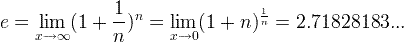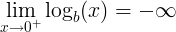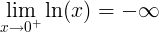# Logarithm and Natural Logarithm

The base b logarithm of a number is the exponent that we need to raise the base in order to get the number.

Logarithm definition

When b is raised to the power of y is equal x:

b y = x

Then the base b logarithm of x is equal to y:

logb(x) = y

For example when:

24 = 16

Then

log2(16) = 4

### Logarithm as inverse function of exponential function

The logarithmic function,

y = logb(x)

is the inverse function of the exponential function,

x = by

So if we calculate the exponential function of the logarithm of x (x>0),

f (f -1(x)) = blogb(x) = x

Or if we calculate the logarithm of the exponential function of x,

f -1(f (x)) = logb(bx) = x

### Natural logarithm (ln)

Natural logarithm is a logarithm to the base e:

ln(x) = loge(x)

When e constant is the number:### Inverse logarithm calculation

The inverse logarithm (or anti logarithm) is calculated by raising the base b to the logarithm y:

x = log-1(y) = b y

### Logarithmic function

The logarithmic function has the basic form of:

f (x) = logb(x)

Logarithm rules

Rule name
Rule
Logarithm product rule
logb(x ∙ y) = logb(x) + logb(y)
Logarithm quotient rule
logb(x / y) = logb(x)logb(y)
Logarithm power rule
logb(x y) = y ∙ logb(x)
Logarithm base switch rule
logb(c) = 1 / logc(b)
Logarithm base change rule
logb(x) = logc(x) / logc(b)
Derivative of logarithm
f (x) = logb(x)⇒ f ‘ (x) = 1 / ( x ln(b) )
Integral of logarithm
∫logb(x) dx = x ∙ ( logb(x)- 1 / ln(b)) + C
Logarithm of negative number
logb(x)is undefined when x≤ 0
Logarithm of 0
logb(0) is undefined
Logarithm of 0Logarithm of 1
logb(1) = 0
Logarithm of the base
logb(b) = 1
Logarithm of infinity
lim logb() = ∞,when x→∞

Logarithm product rule

The logarithm of the multiplication of x and y is the sum of logarithm of x and logarithm of y.

logb(x ∙ y) = logb(x) + logb(y)

For example:

log10(37) = log10(3) + log10(7)

Logarithm quotient rule

The logarithm of the division of x and y is the difference of logarithm of x and logarithm of y.

logb(x / y) = logb(x)logb(y)

For example:

log10(3 / 7) = log10(3)log10(7)

Logarithm power rule

The logarithm of x raised to the power of y is y times the logarithm of x.

logb(x y) = y ∙ logb(x)

For example:

log10(28) = 8log10(2)

Logarithm base switch rule

The base b logarithm of c is 1 divided by the base c logarithm of b.

logb(c) = 1 / logc(b)

For example:

log2(8) = 1 / log8(2)

Logarithm base change rule

The base b logarithm of x is base c logarithm of x divided by the base c logarithm of b.

logb(x) = logc(x) / logc(b)

For example, in order to calculate log2(8) in calculator, we need to change the base to 10:

log2(8) = log10(8) / log10(2)

Logarithm of negative number

The base b real logarithm of x when x<=0 is undefined when x is negative or equal to zero:

logb(x) is undefined when x ≤ 0

Logarithm of 0

The base b logarithm of zero is undefined:

logb(0) is undefined

The limit of the base b logarithm of x, when x approaches zero, is minus infinity:Logarithm of 1

The base b logarithm of one is zero:

logb(1) = 0

For example, teh base two logarithm of one is zero:

log2(1) = 0

Logarithm of infinity

The limit of the base b logarithm of x, when x approaches infinity, is equal to infinity:

lim logb(x) = ∞, when x→∞

Logarithm of the base

The base b logarithm of b is one:

logb(b) = 1

For example, the base two logarithm of two is one:

log2(2) = 1

Logarithm derivative

When

f (x) = logb(x)

Then the derivative of f(x):

f ‘ (x) = 1 / ( x ln(b) )

Logarithm integral

The integral of logarithm of x:

∫logb(x) dx = x ∙ ( logb(x)- 1 / ln(b)) + C

For example:

∫log2(x) dx = x ∙ ( log2(x)- 1 / ln(2)) + C

Natural logarithm is the logarithm to the base e of a number.

Definition of natural logarithm

When

e y = x

Then base e logarithm of x is

ln(x) = loge(x) = y

The e constant or Euler’s number is:

e ≈ 2.71828183

### Ln as inverse function of exponential function

The natural logarithm function ln(x) is the inverse function of the exponential function ex.

For x>0,

f (f -1(x)) = eln(x) = x

Or

f -1(f (x)) = ln(ex) = x

Natural logarithm rules and properties

Rule name
Rule
Example
Product rule
ln(x ∙ y) = ln(x) + ln(y)
ln(37) = ln(3) + ln(7)
Quotient rule
ln(x / y) = ln(x)ln(y)
ln(3 / 7) = ln(3)ln(7)
Power rule
ln(x y) = y ∙ ln(x)
ln(28) = 8ln(2)
ln derivative
f (x) = ln(x)⇒ f ‘ (x) = 1 / x

ln integral
∫ln(x)dx = x ∙ (ln(x) – 1) + C
ln of negative number
ln(x) is undefined when x ≤ 0
ln of zero
ln(0) is undefined
ln of zeroln of one
ln(1) = 0
ln of infinity
lim ln(x) = ∞ ,when x→∞

#### Logarithm product rule

The logarithm of the multiplication of x and y is the sum of logarithm of x and logarithm of y.

logb(x ∙ y) = logb(x) + logb(y)

For example:

log10(37) = log10(3) + log10(7)

Logarithm quotient rule

The logarithm of the division of x and y is the difference of logarithm of x and logarithm of y.

logb(x / y) = logb(x)logb(y)

For example:

log10(3 / 7) = log10(3)log10(7)

Logarithm power rule

The logarithm of x raised to the power of y is y times the logarithm of x.

logb(x y) = y ∙ logb(x)

For example:

log10(28) = 8log10(2)

Derivative of natural logarithm

The derivative of the natural logarithm function is the reciprocal function.

When

f (x) = ln(x)

The derivative of f(x) is:

f ‘ (x) = 1 / x

Integral of natural logarithm

The integral of the natural logarithm function is given by:

When

f (x) = ln(x)

The integral of f(x) is:

f (x)dx = ∫ln(x)dx = x ∙ (ln(x) – 1) + C

Ln of 0

The natural logarithm of zero is undefined:

ln(0) is undefined

The limit near 0 of the natural logarithm of x, when x approaches zero, is minus infinity:Ln of 1

The natural logarithm of one is zero:

ln(1) = 0

Ln of infinity

The limit of natural logarithm of infinity, when x approaches infinity is equal to infinity:

lim ln(x) = ∞, when x→∞